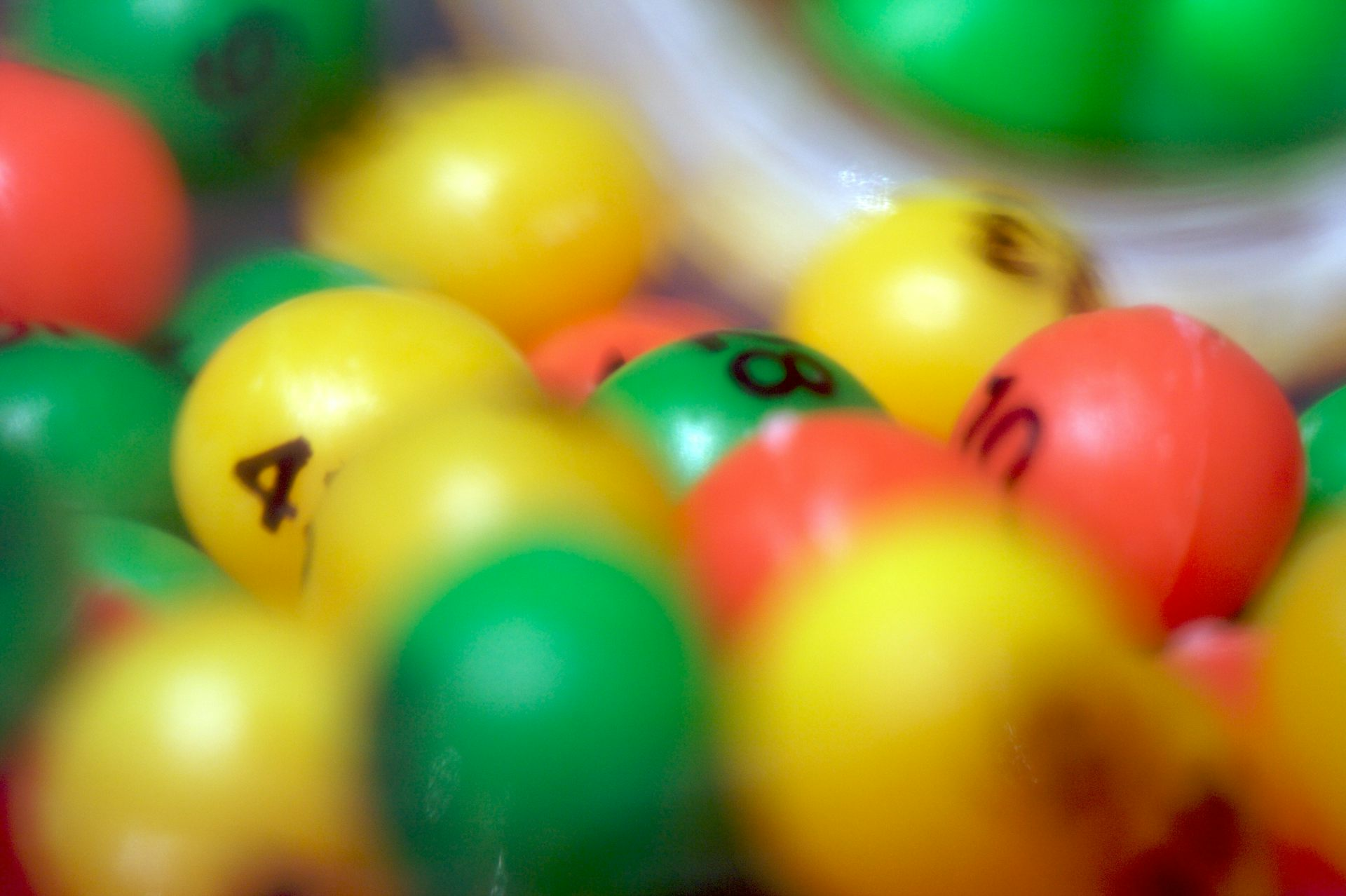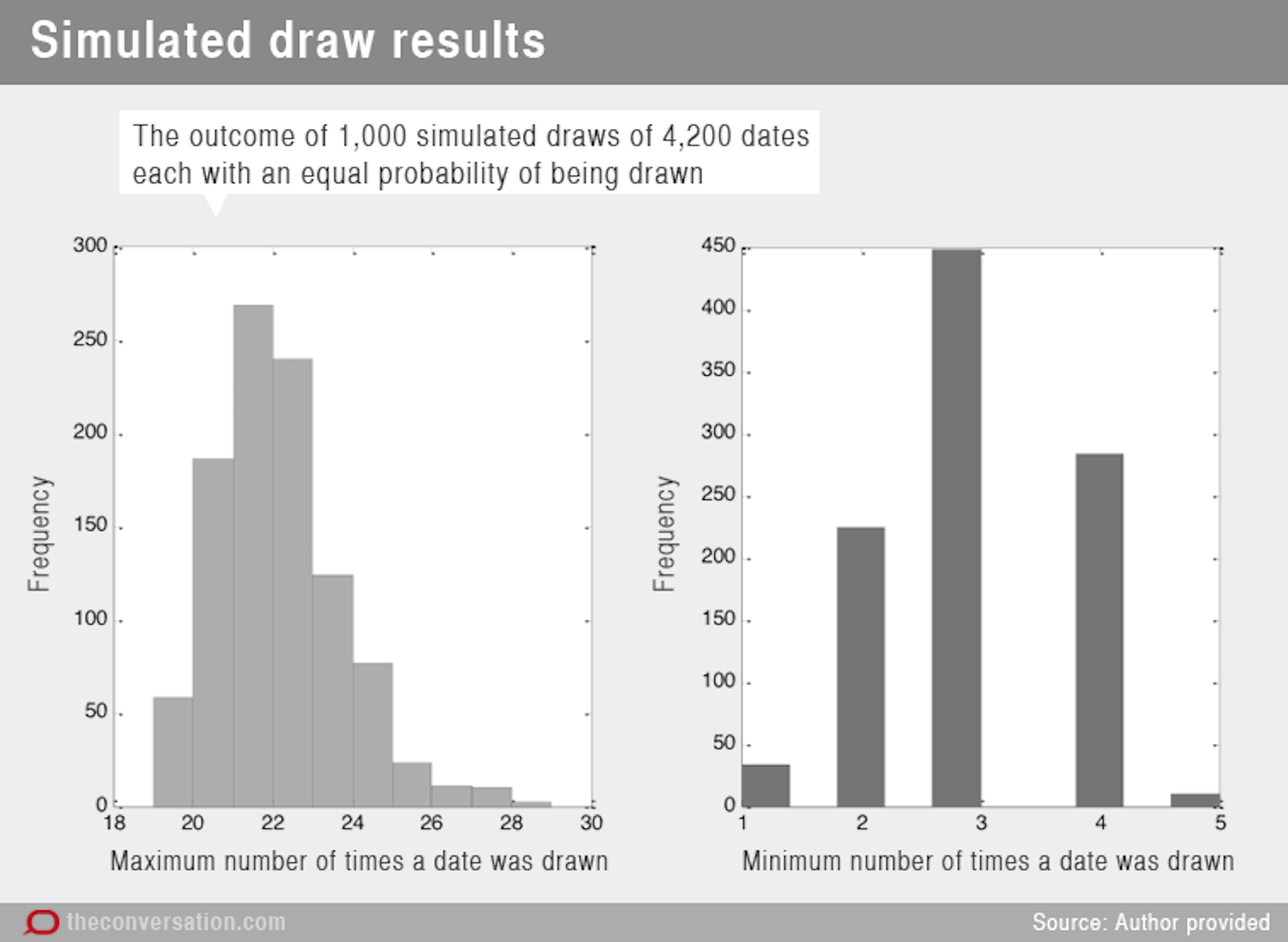# The numbers reveal the government didn't 'play god' with the Vietnam draft

Thursday, Jul 2, 2015, 07:17 AM | Source: The Conversation

Daniel R. Little, Chris DonkinThe conscription ballot was conducted via a lottery, which was supposed to be unbiased towards any particular date. Jeremy Brooks/Flickr, CC BY-NC

Former Deputy Prime Minister Tim Fischer argued today in The Age that public servants “played god” with the Vietnam draft. According to Fischer, they fudged the draw.

He provided a handful of examples to illustrate this claim, including that the geographical locations of the draftees were not uniformly distributed across Australia and that some birth dates were more likely to be drawn than others.

As quoted by David Ellery in The Age:

Only four marbles came up for men born on January 1, 1946, compared to 13 marbles for men born on June 30, 1946. This is almost beyond the standard deviation [you would expect].

As Ellery states, someone born on June 30 of that year was more than three times as likely to be conscripted as a man born on January 1.

But is this evidence that the draw was manipulated?

## Eyeing the bias

The total number of men drafted was 63,740. So is it actually unusual, if we draw 63,740 dates, to find that some days of the year have a high number of draws and other days do not?

The days of the year can be numbered from 1 to 365 (or 366 on a leap year). And since we aim to find the distribution of counts (or draws) for each date, the dates are distributed according to a multinomial distribution.

This is a generalisation of a binomial distribution, which everyone is familiar with through flipping coins. The binomial distribution tells us the probability of flipping a number of heads (or a number of tails) out of a total number of flips, given the probability of flipping a head.

The probability of flipping a head can be thought of as the bias in the coin. In most coin tosses, we’d like to think that coin is fair, and that the probability of flipping a head is the same as flipping a tail (that is, 1 out of 2).

For dates in a year, the multinomial distribution has an analogous concept, which is the probability that any one of the 365 dates is drawn. For the draw to be completely fair, the probability of drawing any one date should be 1 out of 365 (in a non-leap year).

Fischer’s claim can then be considered as follows: what’s the probability of drawing any one date three times more often than another, out of 63,740 draws with an equal probability of drawing each date?

Here is a figure of the dates ordered by their draw frequency. The first thing to note is that most samples are drawn near the same amount of times (around 174 times for 63,740 draws). In this sample, the most often drawn date (September 1, drawn 211 times) is only 1.48 times more likely than the least-drawn date (December 15, drawn 143 times).

However, these two counts are over 5 standard deviations apart. The reason for this is that the standard deviation of the multinomial distribution is the square root of n x p x (1 – p).

In this case, n is large (63,740) and p is small (1/365), so the standard deviation of the multinomial distribution is 13.2. Is it odd that the highest and lowest counts were 5 standard deviations apart? Not really, even for more familiar normally distributed values, the minimum and maximum sampled values will tend to be 4 to 6 standard deviations apart.

So these results are to be expected of a fair draw. In fact, you’d expect the most and the least drawn dates to be even further apart than one standard deviation.

## Is it random?

The difference between the most sampled and least sampled dates gets larger with smaller samples. The Age reports that the aim of the draft was to “quickly raise the strength of the army from 22,500 to 37,500 troops, by calling up 4,200 youths in the last half of 1965 and and 6,900 [later raised to 8,400] every subsequent year”.

If we repeat the above exercise with the first year’s draft amount of 4,200, then the most sampled date (day 365) was sampled 20 times, but the least sampled date (day 103) was only sampled twice. The least sampled date was sampled 10 times less often than the most sampled date, but the probability of sampling each date was again 1 out of 365.

Why the large discrepancy?

Again, it has to do with the standard deviation of the multinomial distribution. Due to the smaller total number of draws, the standard deviation is now equal to 3.39. So the 20 and the 2 draws are again only 5.3 standard deviations apart, which is completely expected under a uniform sampling scheme.

Tim Fischer may have more information than was reported in The Age, so we can’t rule out the possibility that his claim is correct. However, the difference between 13 draws for 20 June 1946 and 4 draws for 1 Jan 1946 is not as suspect as it might seem.

However, this does serve as a good example of how we humans are not very good at identifying randomness when we see it. In fact, our inability to identify randomness is what makes it possible to use statistics to detect instances of election fraud, such as in the 2009 Iranian election.

To provide another example of how our expectations of randomness might be wrong, we can ask how likely is it that the most frequently drawn date comes up 16 (or more) times, and the least frequently drawn date comes up 4 (or fewer) times.

The following graph shows the outcome of 1,000 simulated draws of 4,200 dates, each with an equal probability of being drawn. We plot how often (the frequency) the most drawn date comes up on the left, and the frequency of the least drawn date on the right.

If anything, we should expect that most and least drawn dates should be more different than they actually were. Again, our expectations for what is random do not reflect the underlying true outcomes from random sampling.Daniel Little receives funding from Australia Research Council Discovery Project grants.

Chris Donkin receives funding from Australian Research Council Discovery Project grants.